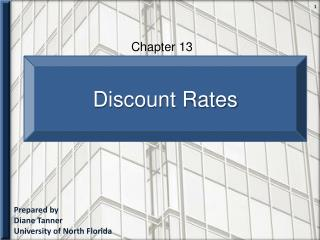DownloadDownload PresentationPrepared by Diane Tanner University of North Florida

# Prepared by Diane Tanner University of North Florida

Télécharger la présentation## Prepared by Diane Tanner University of North Florida

- - - - - - - - - - - - - - - - - - - - - - - - - - - E N D - - - - - - - - - - - - - - - - - - - - - - - - - - -
##### Presentation Transcript

1. Chapter 13 Discount Rates Prepared by Diane Tanner University of North Florida

2. What is a Discount Rate? • An interest rate • Used to determine the cost of money over time • Its use in managerial accounting decisions • To determine how much future cash flow amounts are worth in the present • Two common discount rates • Weighted average cost of capital • Referred to as the 'cost of capital' or WACC • Required rate of return (RRR) • When assessing capital budget decisions • Use the required rate of return

3. Cost of Capital Assets are ‘financed’ by the two equities on the right side of the accounting equation Assets = Liabilities + Owners’ Equity Equity Financing Debt Financing • Occurs when a company • Obtains long-term loans /bonds • Occurs when a company • Issues stock • Earns profit which increases net worth

4. Cost of Capital Assets = Liabilities + Owners’ Equity Debt Financing Equity Financing Creates interest expense which reduces profit • Creates a cost consisting of returns to investors • Dividends • Stock value growth thru earnings retention Managers weigh the two costs based on the proportion of the company which is financed by debt and the proportion financed by equity financing. Weighted average cost of capital Results in

5. Required Rate of Return (RRR) • Weighted average cost of capital Required Rate of Return = • Sometimes a % component for desired profit is added • Also known as the hurdle rate • Indicates the minimum amount—the hurdle—an investment must meet in order to be accepted • + Risk % + Inflation %

6. The End